# 1. statistics统计计算

statistics模块实现了很多常用的统计公式，允许使用Python的各种数值类型(int、float、Decimal和Fraction)来完成高效计算。

## 1.1 平均值

from statistics import *

data = [1, 2, 2, 5, 10, 12]

print('{:0.2f}'.format(mean(data)))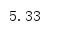from statistics import *

data = [1, 2, 2, 5, 10, 12]

print(mode(data))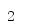from statistics import *

data = [1, 2, 2, 5, 10, 12]

print('median     : {:0.2f}'.format(median(data)))
print('low        : {:0.2f}'.format(median_low(data)))
print('high       : {:0.2f}'.format(median_high(data)))

median()会查找中间的值。如果数据集包含偶数个值，则取两个中间元素的平均值。median_low()总是返回输入数据集中的一个值，对于有偶数个元素的数据集，会返回两个中间元素中较小的一个。median_high()与之类似，不过会返回两个中间元素中较大的一个。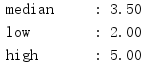from statistics import *

data = [10, 20, 30, 40]

print('1: {:0.2f}'.format(median_grouped(data, interval=1)))
print('2: {:0.2f}'.format(median_grouped(data, interval=2)))
print('3: {:0.2f}'.format(median_grouped(data, interval=3)))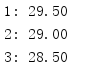## 1.2 方差

import statistics

x =[1,2,3,4,5,10,9,8,7,6]
y1 = statistics.pvariance(x)
print(y1)
y2 = statistics.pstdev(x)
print(y2)
y3 = statistics.variance(x)
print(y3)
y4 = statistics.stdev(x)
print(y4)

Python包括两组函数来计算方差和标准差，具体取决于数据集是表示总体还是总体中的一个样本。这个例子首先pvariance()和pstdev()计算总体的方差和标准哈。然后使用variance()和stdev()计算一个子集的样本方差和标准差。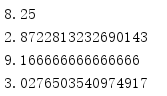posted @ 2020-03-10 16:14  SmallGrayCode  阅读(1768)  评论(0编辑  收藏  举报